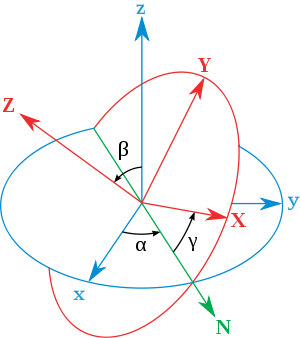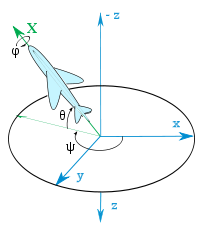# Conversion between quaternions and Euler angles

Conversion between quaternions and Euler angles

Spatial rotations in three dimensions can be parametrized using both Euler angles and unit quaternions. This article explains how to convert between the two representations. Actually this simple use of "quaternions" was first presented by Euler some seventy years earlier than Hamilton to solve the problem of magic squares. For this reason the dynamics community commonly refers to quaternions in this application as "Euler parameters".

## Definition

A unit quaternion can be described as:$\mathbf{q} = \begin{bmatrix} q_0 & q_1 & q_2 & q_3 \end{bmatrix}^T$$|\mathbf{q}|^2 = q_0^2 + q_1^2 + q_2^2 + q_3^2 = 1$

We can associate a quaternion with a rotation around an axis by the following expression$\mathbf{q}_0 = \cos(\alpha/2)$$\mathbf{q}_1 = \sin(\alpha/2)\cos(\beta_x)$$\mathbf{q}_2 = \sin(\alpha/2)\cos(\beta_y)$$\mathbf{q}_3 = \sin(\alpha/2)\cos(\beta_z)$

where α is a simple rotation angle (the value in radians of the angle of rotation) and cos(βx), cos(βy) and cos(βz) are the "direction cosines" locating the axis of rotation (Euler's Theorem).

## Rotation matricesEuler angles – The xyz (fixed) system is shown in blue, the XYZ (rotated) system is shown in red. The line of nodes, labelled N, is shown in green.

The orthogonal matrix (post-multiplying a column vector) corresponding to a clockwise/left-handed rotation by the unit quaternion q = q0 + iq1 + jq2 + kq3 is given by the inhomogeneous expression$\begin{bmatrix} 1- 2(q_2^2 + q_3^2) & 2(q_1 q_2 - q_0 q_3) & 2(q_0 q_2 + q_1 q_3) \\ 2(q_1 q_2 + q_0 q_3) & 1 - 2(q_1^2 + q_3^2) & 2(q_2 q_3 - q_0 q_1) \\ 2(q_1 q_3 - q_0 q_2) & 2( q_0 q_1 + q_2 q_3) & 1 - 2(q_1^2 + q_2^2) \end{bmatrix}$

or equivalently, by the homogeneous expression$\begin{bmatrix} q_0^2 + q_1^2 - q_2^2 - q_3^2 & 2(q_1 q_2 - q_0 q_3) & 2(q_0 q_2 + q_1 q_3) \\ 2(q_1 q_2 + q_0 q_3) & q_0^2 - q_1^2 + q_2^2 - q_3^2 & 2(q_2 q_3 - q_0 q_1) \\ 2(q_1 q_3 - q_0 q_2) & 2( q_0 q_1 + q_2 q_3) & q_0^2 - q_1^2 - q_2^2 + q_3^2 \end{bmatrix}$

If q0 + iq1 + jq2 + kq3 is not a unit quaternion then the homogeneous form is still a scalar multiple of a rotation matrix, while the inhomogeneous form is in general no longer an orthogonal matrix. This is why in numerical work the homogeneous form is to be preferred if distortion is to be avoided.

The orthogonal matrix (post-multiplying a column vector) corresponding to a clockwise/left-handed rotation with Euler angles φ, θ, ψ, with x-y-z convention, is given by:$\begin{bmatrix} \cos\theta \cos\psi & -\cos\phi \sin\psi + \sin\phi \sin\theta \cos\psi & \sin\phi \sin\psi + \cos\phi \sin\theta \cos\psi \\ \cos\theta \sin\psi & \cos\phi \cos\psi + \sin\phi \sin\theta \sin\psi & -\sin\phi \cos\psi + \cos\phi \sin\theta \sin\psi \\ -\sin\theta & \sin\phi \cos\theta & \cos\phi \cos\theta \\ \end{bmatrix}$

### Conversion

By combining the quaternion representations of the Euler rotations we get$\mathbf{q} = R_z(\psi)R_y(\theta)R_x(\phi) = [\cos (\psi /2) + \mathbf{k}\sin (\psi /2)][\cos (\theta /2) + \mathbf{j}\sin (\theta /2)][\cos (\phi /2) + \mathbf{i}\sin (\phi /2)]$$\mathbf{q} = \begin{bmatrix} \cos (\phi /2) \cos (\theta /2) \cos (\psi /2) + \sin (\phi /2) \sin (\theta /2) \sin (\psi /2) \\ \sin (\phi /2) \cos (\theta /2) \cos (\psi /2) - \cos (\phi /2) \sin (\theta /2) \sin (\psi /2) \\ \cos (\phi /2) \sin (\theta /2) \cos (\psi /2) + \sin (\phi /2) \cos (\theta /2) \sin (\psi /2) \\ \cos (\phi /2) \cos (\theta /2) \sin (\psi /2) - \sin (\phi /2) \sin (\theta /2) \cos (\psi /2) \\ \end{bmatrix}$

For Euler angles we get:$\begin{bmatrix} \phi \\ \theta \\ \psi \end{bmatrix} = \begin{bmatrix} \mbox{arctan} \frac {2(q_0 q_1 + q_2 q_3)} {1 - 2(q_1^2 + q_2^2)} \\ \mbox{arcsin} (2(q_0 q_2 - q_3 q_1)) \\ \mbox{arctan} \frac {2(q_0 q_3 + q_1 q_2)} {1 - 2(q_2^2 + q_3^2)} \end{bmatrix}$

arctan and arcsin have a result between −π/2 and π/2. With three rotation between −π/2 and π/2 you can't have all possible orientations. You need to replace the arctan by atan2 to generate all the orientation.$\begin{bmatrix} \phi \\ \theta \\ \psi \end{bmatrix} = \begin{bmatrix} \mbox{atan2} (2(q_0 q_1 + q_2 q_3),1 - 2(q_1^2 + q_2^2)) \\ \mbox{arcsin} (2(q_0 q_2 - q_3 q_1)) \\ \mbox{atan2} (2(q_0 q_3 + q_1 q_2),1 - 2(q_2^2 + q_3^2)) \end{bmatrix}$

## Relationship with Tait–Bryan anglesTait–Bryan angles for an aircraft

Similarly for Euler angles, we use the Tait–Bryan angles (in terms of flight dynamics):

• Roll – ϕ: rotation about the X-axis
• Pitch – θ: rotation about the Y-axis
• Yaw – ψ: rotation about the Z-axis

where the X-axis points forward, Y-axis to the right and Z-axis downward and in the example to follow the rotation occurs in the order yaw, pitch, roll (about body-fixed axes).

## Singularities

One must be aware of singularities in the Euler angle parametrization when the pitch approaches ±90° (north/south pole). These cases must be handled specially. The common name for this situation is gimbal lock.

Code to handle the singularities is derived on this site: www.euclideanspace.com

Wikimedia Foundation. 2010.

### Look at other dictionaries:

• Euler angles — The Euler angles were developed by Leonhard Euler to describe the orientation of a rigid body (a body in which the relative position of all its points is constant) in 3 dimensional Euclidean space. To give an object a specific orientation it may… …   Wikipedia

• Quaternions and spatial rotation — Unit quaternions provide a convenient mathematical notation for representing orientations and rotations of objects in three dimensions. Compared to Euler angles they are simpler to compose and avoid the problem of gimbal lock. Compared to… …   Wikipedia

• Rotation representation (mathematics) — In geometry a rotation representation expresses the orientation of an object (or coordinate frame) relative to a coordinate reference frame. This concept extends to classical mechanics where rotational (or angular) kinematics is the science of… …   Wikipedia

• Dual quaternion — The set of dual quaternions is an algebra that can be used to represent spatial rigid body displacements. A dual quaternion is an ordered pair of quaternions Â = (A, B) and therefore is constructed from eight real parameters. Because rigid… …   Wikipedia

• List of mathematics articles (C) — NOTOC C C closed subgroup C minimal theory C normal subgroup C number C semiring C space C symmetry C* algebra C0 semigroup CA group Cabal (set theory) Cabibbo Kobayashi Maskawa matrix Cabinet projection Cable knot Cabri Geometry Cabtaxi number… …   Wikipedia

• Quaternion — Quaternions, in mathematics, are a non commutative extension of complex numbers. They were first described by the Irish mathematician Sir William Rowan Hamilton in 1843 and applied to mechanics in three dimensional space. They find uses in both… …   Wikipedia

• Rotation matrix — In linear algebra, a rotation matrix is a matrix that is used to perform a rotation in Euclidean space. For example the matrix rotates points in the xy Cartesian plane counterclockwise through an angle θ about the origin of the Cartesian… …   Wikipedia

• logic, history of — Introduction       the history of the discipline from its origins among the ancient Greeks to the present time. Origins of logic in the West Precursors of ancient logic       There was a medieval tradition according to which the Greek philosopher …   Universalium